# AND Gate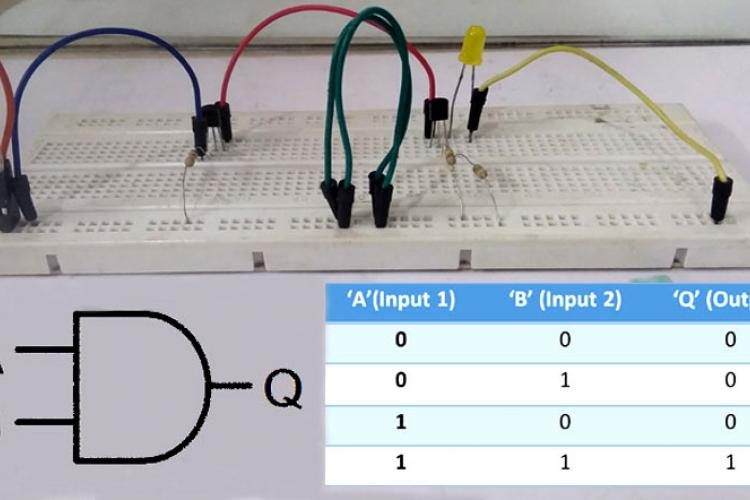Designing an AND Gate using TransistorsAs many of us know an Integrated Circuit or IC is a combination of many small circuits in a small package which together…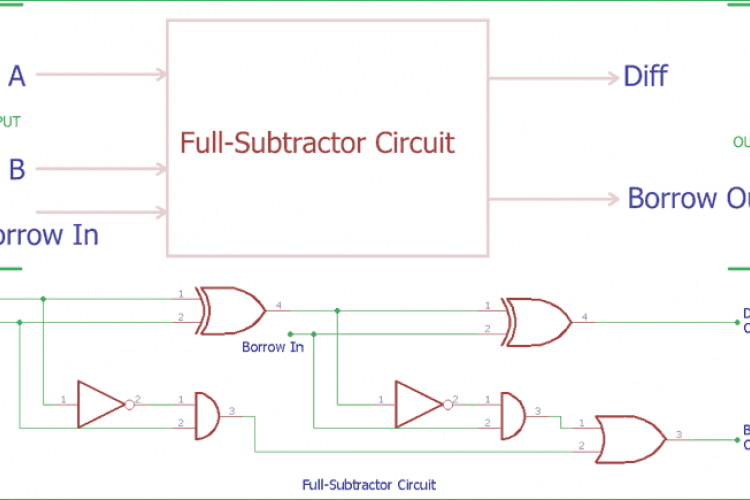Full Subtractor Circuit and Its ConstructionIn the previous tutorial of Half Subtractor Circuit, we had seen how computer use single bit binary numbers 0 and 1 for…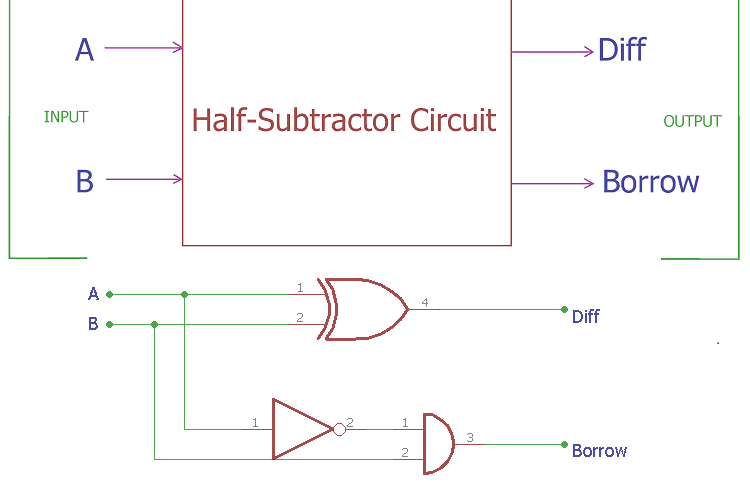Half Subtractor Circuit and Its ConstructionIn previous tutorials, we have seen how computer use binary numbers 0 and 1 and by using an adder circuit computer will add…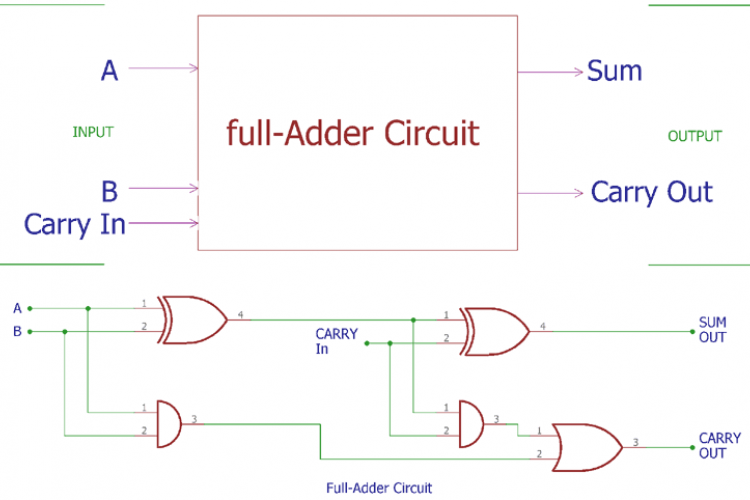Full Adder Circuit and its ConstructionIn previous tutorial of half adder circuit construction, we had seen how computer uses single bit binary numbers 0 and 1 for…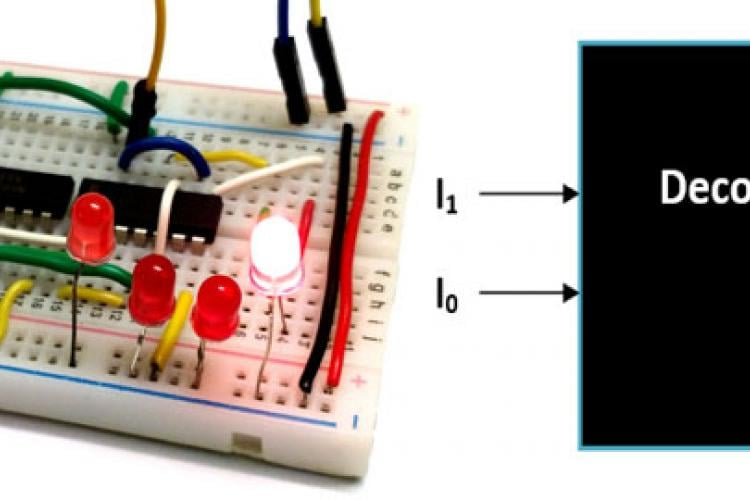Binary DecodersDecoder is type of combinational Circuit which decodes a small bit value into large bit value. It is normally used in…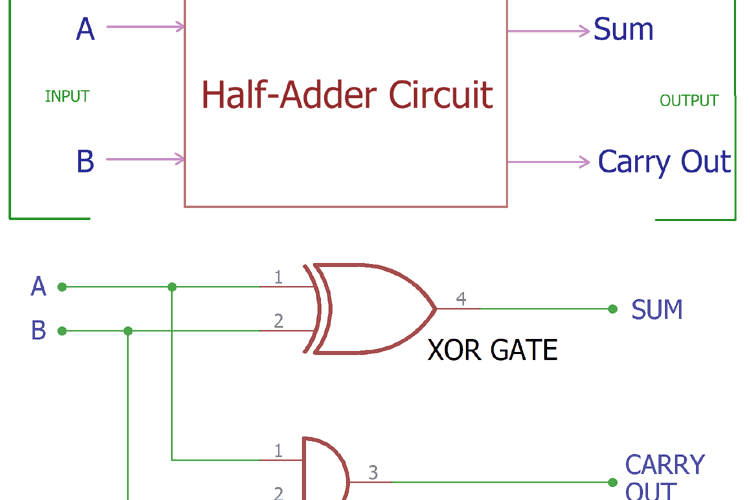Half Adder Circuit and its ConstructionComputer uses binary numbers 0 and 1. An adder circuit uses these binary numbers and calculates the addition. A binary adder…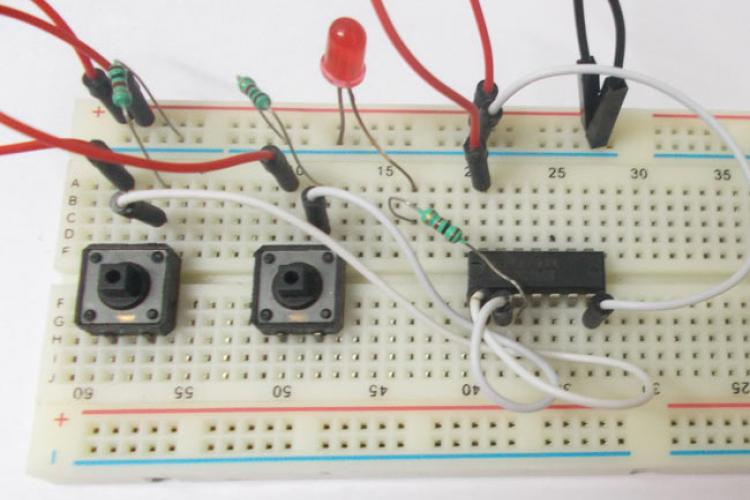AND Gate CircuitAND logic gate is a digital logic gate, designed for arithmetic and logical operations, every electronic student must have…